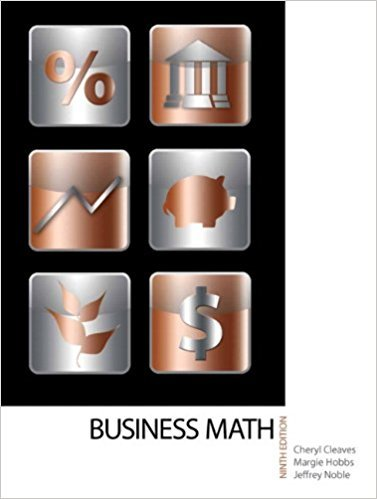×
×

# Solutions for Chapter 3-2: OPERATIONS WITH DECIMALS## Full solutions for Business Math, | 9th Edition

ISBN: 9780135108178Solutions for Chapter 3-2: OPERATIONS WITH DECIMALS

Solutions for Chapter 3-2
4 5 0 361 Reviews
13
5
##### ISBN: 9780135108178

Since 30 problems in chapter 3-2: OPERATIONS WITH DECIMALS have been answered, more than 17372 students have viewed full step-by-step solutions from this chapter. Chapter 3-2: OPERATIONS WITH DECIMALS includes 30 full step-by-step solutions. This textbook survival guide was created for the textbook: Business Math, , edition: 9. Business Math, was written by and is associated to the ISBN: 9780135108178. This expansive textbook survival guide covers the following chapters and their solutions.

Key Math Terms and definitions covered in this textbook
• Augmented matrix [A b].

Ax = b is solvable when b is in the column space of A; then [A b] has the same rank as A. Elimination on [A b] keeps equations correct.

• Block matrix.

A matrix can be partitioned into matrix blocks, by cuts between rows and/or between columns. Block multiplication ofAB is allowed if the block shapes permit.

• Condition number

cond(A) = c(A) = IIAIlIIA-III = amaxlamin. In Ax = b, the relative change Ilox III Ilx II is less than cond(A) times the relative change Ilob III lib II· Condition numbers measure the sensitivity of the output to change in the input.

• Eigenvalue A and eigenvector x.

Ax = AX with x#-O so det(A - AI) = o.

• Ellipse (or ellipsoid) x T Ax = 1.

A must be positive definite; the axes of the ellipse are eigenvectors of A, with lengths 1/.JI. (For IIx II = 1 the vectors y = Ax lie on the ellipse IIA-1 yll2 = Y T(AAT)-1 Y = 1 displayed by eigshow; axis lengths ad

• Exponential eAt = I + At + (At)2 12! + ...

has derivative AeAt; eAt u(O) solves u' = Au.

• Four Fundamental Subspaces C (A), N (A), C (AT), N (AT).

Use AT for complex A.

• Full row rank r = m.

Independent rows, at least one solution to Ax = b, column space is all of Rm. Full rank means full column rank or full row rank.

• Indefinite matrix.

A symmetric matrix with eigenvalues of both signs (+ and - ).

• Independent vectors VI, .. " vk.

No combination cl VI + ... + qVk = zero vector unless all ci = O. If the v's are the columns of A, the only solution to Ax = 0 is x = o.

• Iterative method.

A sequence of steps intended to approach the desired solution.

• Kirchhoff's Laws.

Current Law: net current (in minus out) is zero at each node. Voltage Law: Potential differences (voltage drops) add to zero around any closed loop.

• Nilpotent matrix N.

Some power of N is the zero matrix, N k = o. The only eigenvalue is A = 0 (repeated n times). Examples: triangular matrices with zero diagonal.

• Nullspace N (A)

= All solutions to Ax = O. Dimension n - r = (# columns) - rank.

• Orthogonal subspaces.

Every v in V is orthogonal to every w in W.

• Projection p = a(aTblaTa) onto the line through a.

P = aaT laTa has rank l.

• Row space C (AT) = all combinations of rows of A.

Column vectors by convention.

• Schur complement S, D - C A -} B.

Appears in block elimination on [~ g ].

• Similar matrices A and B.

Every B = M-I AM has the same eigenvalues as A.

• Triangle inequality II u + v II < II u II + II v II.

For matrix norms II A + B II < II A II + II B II·

×